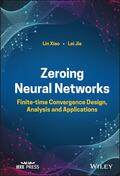John Wiley & Sons Zeroing Neural NetworksZeroing Neural Networks Describes the theoretical and practical aspects of finite-time ZNN methods .. Product #: 978-1-119-98599-0 Regular price: \$120.56 \$120.56 In Stock

# Zeroing Neural Networks

## Finite-time Convergence Design, Analysis and Applications1. Edition November 2022
432 Pages, Hardcover
Wiley & Sons Ltd

ISBN: 978-1-119-98599-0
John Wiley & Sons

### Price: 129,00 €

Price incl. VAT, excl. Shipping

Zeroing Neural Networks

Describes the theoretical and practical aspects of finite-time ZNN methods for solving an array of computational problems

Zeroing Neural Networks (ZNN) have become essential tools for solving discretized sensor-driven time-varying matrix problems in engineering, control theory, and on-chip applications for robots. Building on the original ZNN model, finite-time zeroing neural networks (FTZNN) enable efficient, accurate, and predictive real-time computations. Setting up discretized FTZNN algorithms for different time-varying matrix problems requires distinct steps.

Zeroing Neural Networks provides in-depth information on the finite-time convergence of ZNN models in solving computational problems. Divided into eight parts, this comprehensive resource covers modeling methods, theoretical analysis, computer simulations, nonlinear activation functions, and more. Each part focuses on a specific type of time-varying computational problem, such as the application of FTZNN to the Lyapunov equation, linear matrix equation, and matrix inversion. Throughout the book, tables explain the performance of different models, while numerous illustrative examples clarify the advantages of each FTZNN method. In addition, the book:
* Describes how to design, analyze, and apply FTZNN models for solving computational problems
* Presents multiple FTZNN models for solving time-varying computational problems
* Details the noise-tolerance of FTZNN models to maximize the adaptability of FTZNN models to complex environments
* Includes an introduction, problem description, design scheme, theoretical analysis, illustrative verification, application, and summary in every chapter

Zeroing Neural Networks: Finite-time Convergence Design, Analysis and Applications is an essential resource for scientists, researchers, academic lecturers, and postgraduates in the field, as well as a valuable reference for engineers and other practitioners working in neurocomputing and intelligent control.

LIN XIAO, PhD, is a Professor in the College of Information Science and Engineering at Hunan Normal University, Changsha, China. He has authored more than 100 papers in international conferences and journals, including IEEE-TCYB, IEEE-TII, IEEE-TSMCS. Professor Xiao is Associate Editor of IEEE-TNNLS.

LEI JIA is a PhD degree candidate in Operations Research and Control in the College of Mathematics and Statistics at Hunan Normal University, Changsha, China. She has authored or co-authored more than 20 scientific articles, including 13 IEEE-transaction papers.